C++ OOP

1.概述

2.继承

2.0 基类

2.1 派生类

2.2 空基类优化（本节不理解

2.3 虚基类

2.4 继承方式

2.5 成员名称查找（不理解）

2.6 C++11新增

override

final

3. 动态绑定

3.1 对象模型：虚表和虚指针

3.2 动态绑定的三个条件

3.3 static type和dynamic type区别

3.4 关于this

4. 虚成员、虚函数

4.1 定义

4.2 调用（重要）

4.3 覆写（重要）

4.4 协变返回类型

4.5 在构造与析构期间

5. 抽象类 与 纯虚类

1.概述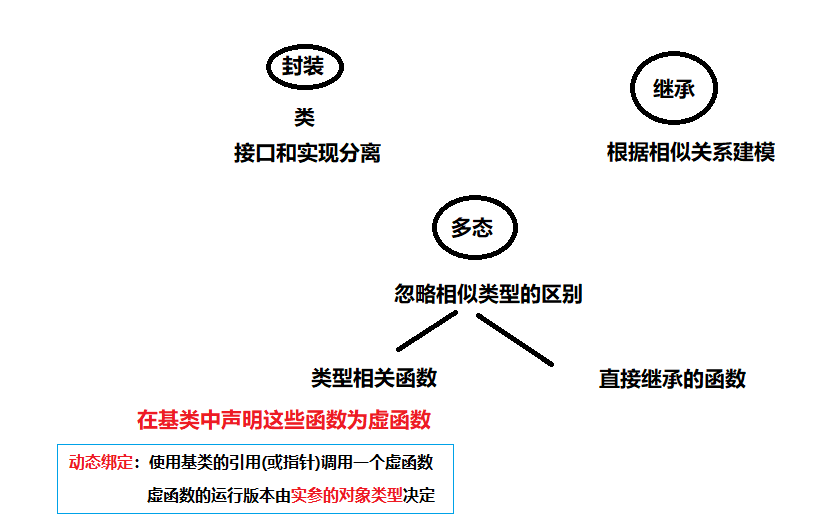2.继承

struct Base {
int a, b, c;
};
// 每个 Derived 类型对象包含 Base 为子对象
struct Derived : Base {
int b;
};
// 每个 Derived2 类型对象包含 Derived 与 Base 为子对象
struct Derived2 : Derived {
int c;
};
• 从内存的角度：派生类继承了基类的成员变量（data)。
• 从多态的角度：可以重写基类的成员函数。函数的继承是继承父类的调用权

2.0 基类

• non-virtual fun 非虚函数：不希望派生类重写。
• virtual fun 虚函数 ：希望派生类重写。
• pure virtual fun 纯虚函数 ： 派生类必须重写。

class Base {
public:
virtual ~Base() { /* 释放 Base 的资源 */ }
};

class Derived : public Base {
~Derived() { /* 释放 Derived 的资源 */ }
};

int main()
{
Base* b = new Derived;
delete b; // 进行到 Base::~Base() 的虚函数调用
// 因为它为虚，故它调用 Derived::~Derived() ，
// 能释放派生类的资源，然后遵循通常析构顺序
// 调用 Base::~Base()
}

【重要！】任何基类的析构函数必须为公开且虚public virtual，或protected受保护且非虚

2.1 派生类

• 派生类必须用 ：指定父类。 用逗号分开。基类前面可以加三种访问说明符之一。
•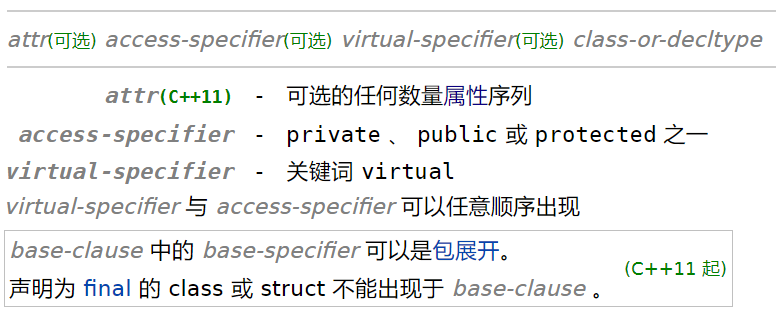• 若省略访问说明符 ，则它对以类关键 struct 声明的类默认为 public ，对以类关键 class 声明的类为 private 。
• 列于 base-clause 的类是直接基类，其基类是间接基类。
• 同一类不能指定于直接基类多于一次，但同一类可以既是直接又是间接基类。
• 每个直接和间接基类都作为基类子对象，以实现定义的偏移存在于派生类的对象表示中。
• 因为空基类优化，空基类通常不会增加派生类对象的大小。
• 类不能派生自己。
• 派生类声明中。不能包含基类列表。
• 派生类可以隐式转换为基类。

2.2 空基类优化（本节不理解

#include <cassert>

struct Base {}; // 空类

struct Derived1 : Base {
int i;
};

int main()
{
// 任何空类类型的对象大小至少为 1
assert(sizeof(Base) > 0);

// 应用空基优化
assert(sizeof(Derived1) == sizeof(int));
}

#include <cassert>

struct Base {}; // 空类

struct Derived1 : Base {
int i;
};

struct Derived2 : Base {
Base c; // Base ，占用 1 字节，后随为 i 的填充
int i;
};

struct Derived3 : Base {
Derived1 c; // 从 Base 派生，占用 sizeof(int) 字节
int i;
};

int main()
{
// 不应用空基优化
// 基类占用 1 字节，Base 成员占用 1 字节，后随2个填充字节以满足 int 对齐要求
assert(sizeof(Derived2) == 2*sizeof(int));

// 不应用空基类优化，
// 基类占用至少 1 字节加填充以满足首个成员的对齐要求（其对齐要求同 int ）
assert(sizeof(Derived3) == 3*sizeof(int));
}

C++11：对于标准布局类型 (StandardLayoutType) 要求有空基类优化，以维持指向标准布局对象的指针，用 reinterpret_cast 转换后，还指向其首成员，这是标准布局类型“无拥有非静态数据成员的基类，且无与其首个非静态数据成员同类型的基类”的原因。

2.3 虚基类

【注意】对于每个指定为 virtual 的相异基类，最终派生类对象仅含有该类型的一个基类子对象，即使该类在继承层级中出现多次（只要它每次都以 virtual 继承）。

struct B { int n; };
class X : public virtual B {};//相异基类
class Y : virtual public B {};//相异基类
class Z : public B {};
// 每个 AA 类型对象拥有一个 X ，一个 Y ，一个 Z 和二个 B ：
// 其一是 Z 的基类，另一者为 X 与 Y 所共享
struct AA : X, Y, Z {
void f() {
X::n = 1; // 修改虚 B 基类子对象的成员
Y::n = 2; // 修改同一虚 B 基类子对象的成员
Z::n = 3; // 修改非虚 B 基类子对象的成员

std::cout << X::n << Y::n << Z::n << '\n'; // 打印 223
}
};

【例子： iostream 】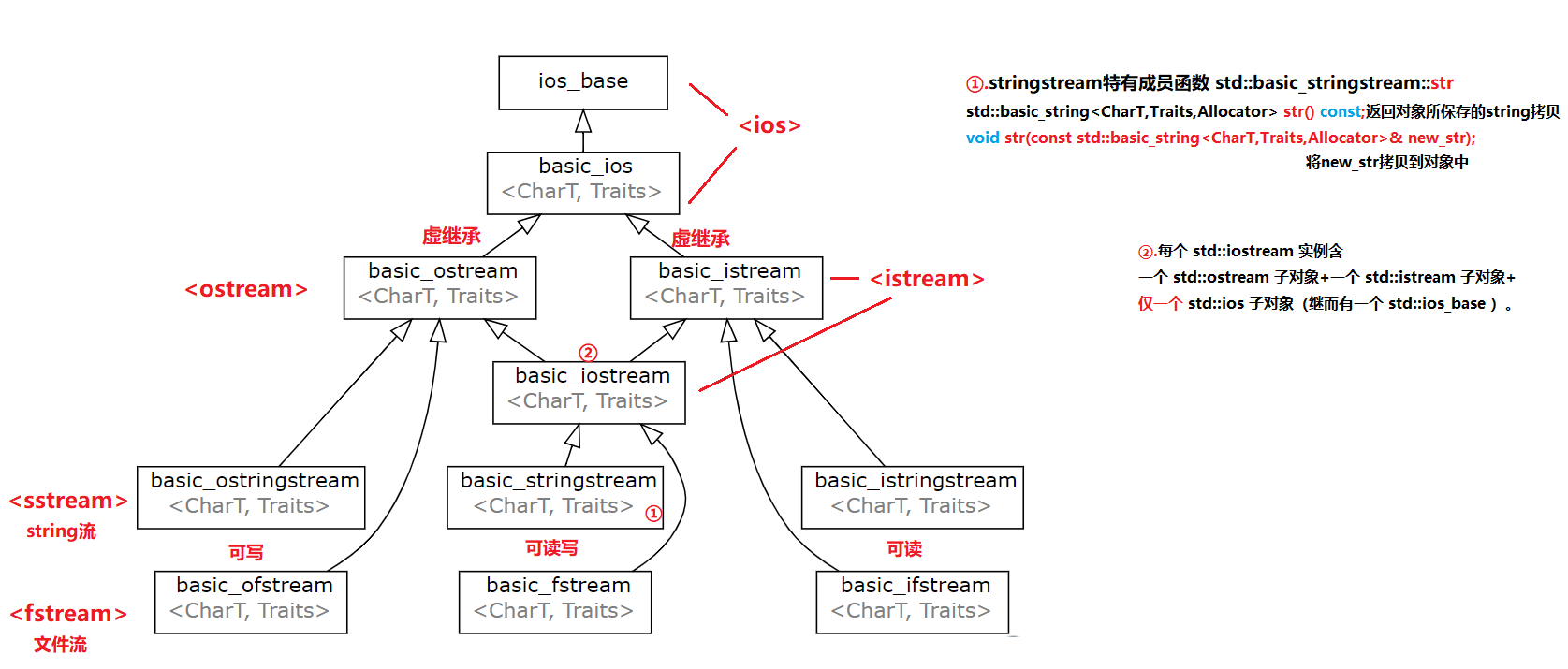std::istream 与 std::ostream 从 std::ios 使用虚继承派生。

（这里不理解）

struct B {
int n;
B(int x) : n(x) {}
};
struct X : virtual B
{
X() : B(1) {}
};
struct Y : virtual B {
Y() : B(2) {}
};
struct AA : X, Y{
AA() : B(3),X(),Y() {} //逗号分隔基类列表。前面可以有访问说明。
};

// AA 的默认构造函数调用 X 和 Y 的默认构造函数
// 但这些构造函数不调用 B 的构造函数，因为 B 是虚基类
AA a; // a.n == 3
// X 的默认构造函数调用 B 的构造函数
X x; // x.n == 1

2.4 继承方式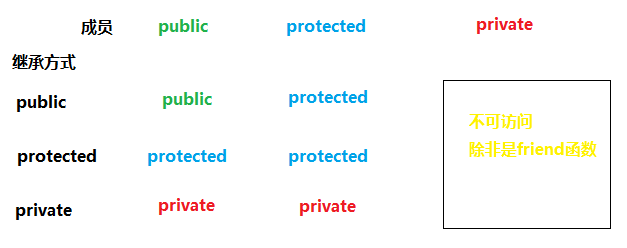template<typename Transport>
class service : Transport  // 从 Transport 策略私有继承
{
public:
void transmit() {
this->send(...);  // 发送传输所提供的任何内容
}
};
// TCP 传输策略
class tcp {
public:
void send(...);
};
// UDP 传输策略
class udp {
public:
void send(...);
};

service<tcp> service(host, port);
service.transmit(...); // 发送完毕 TCP

2.6 C++11新增

override

struct A
{
virtual void foo();
void bar();
};

struct B : A
{
void foo() const override; // 错误： B::foo 不覆写 A::foo
// （签名不匹配）
void foo() override; // OK ： B::foo 覆写 A::foo
void bar() override; // 错误： A::bar 非虚
};

final

final 亦可用于联合体定义，此情况下它无效（除了 std::is_final 上的结果），因为不能派生联合体。final 是在用于成员函数声明或类头部时有特殊含义的标识符。其他语境中它非保留而且可用于命名对象或函数。

struct Base
{
virtual void foo();
};

struct A : Base
{
void foo() final; // A::foo 被覆写且是最终覆写
void bar() final; // 错误：非虚函数不能被覆写或是 final
};

struct B final : A // struct B 为 final
{
void foo() override; // 错误： foo 不能被覆写，因为它在 A 中是 final
};

struct C : B // 错误： B 为 final
{
};

3. 动态绑定(非常重要）

C++ OOP的关键：基类和派生类之间的类型转换

3.1 对象模型：虚表和虚指针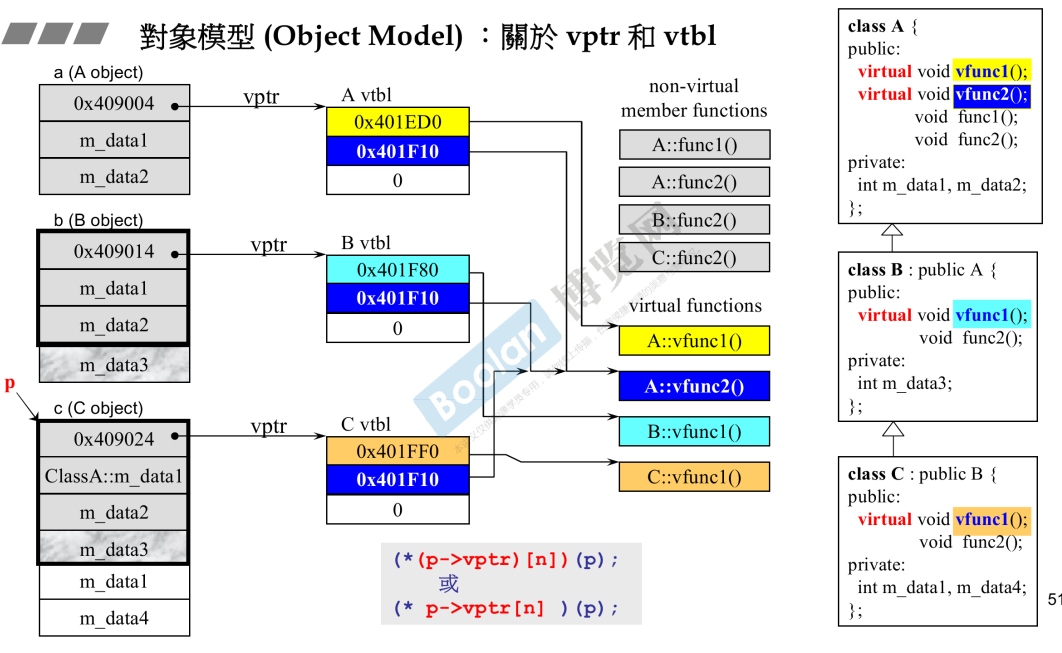•  函数也占内存，也有地址。虚函数才有虚指针，虚函数表（里面都是指向函数的指针。
•  c调用： 静态绑定 call+地址。c++调用：动态绑定。指向C的指针p想调用虚函数v1（动态绑定）通过指针找到vptr虚指针，找到vtbl虚函数表，得到要调用的函数地址。
•  p->vptr[n]是c语言的描述，n是虚函数在vtbl中的第几个位置。编译器在编译的时候看vfun是第几个出现的，就确定了n的值。
• 类的内存：父类数据+自己数据+1个或0个虚指针。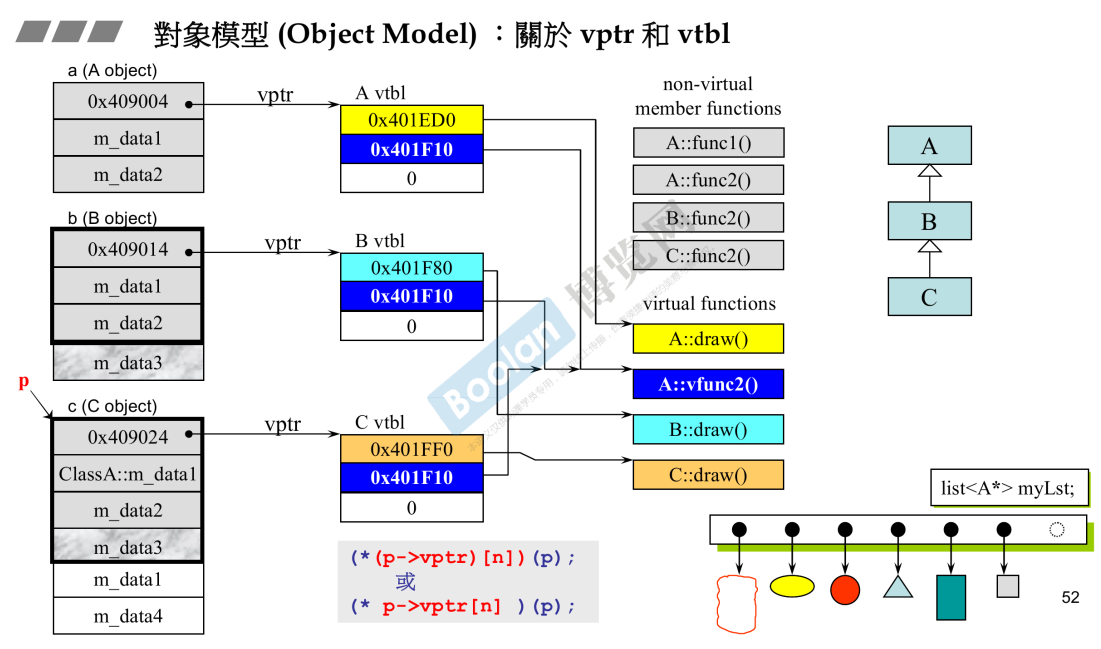• 容器里装的一定是一个指向父类的指针。list<A*>，因为没法确定形状的大小所以是指针，而且必须得是父类。
• 只有虚函数才能被override（c++)。不用像c那样去判断类型。因为父类可能加新的子类。

3.2 动态绑定的三个条件

1. 通过指针或引用调用。
2. 指针必须向上转型。由子类转向父类。
3. 调用的是虚函数。

3.3 static type和dynamic type区别

• 静态类型在编译时就被确定了。它是【变量声明时的类型】 或【表达式生成的类型】。
• 动态类型在运行时才知道。它是【变量或表达式代表的内存中的对象的类型】。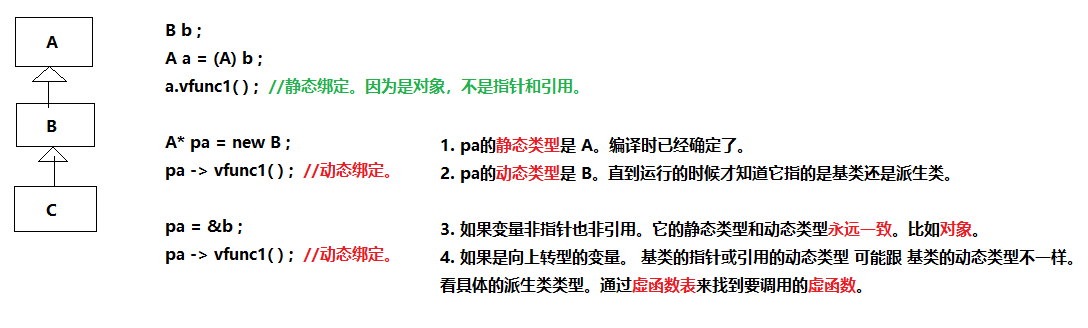3.4 关于this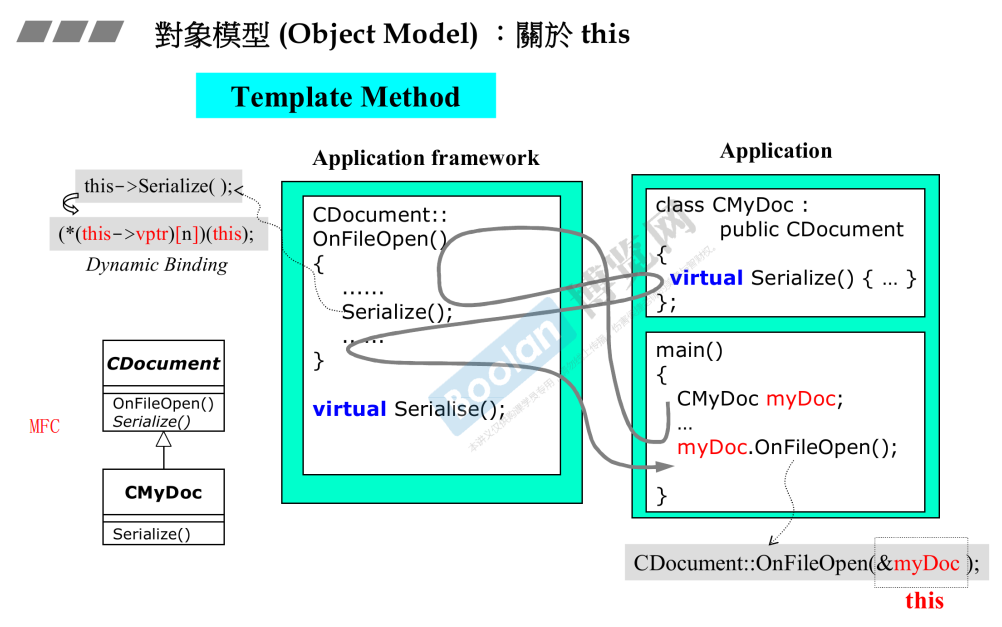•  this是个指针。也可以说this指的那个object。
• main(){derivedclass object;object.func();}相当于调用baseclass::func(&object);&object就是this，通常不写。

4. 虚成员、虚函数

4.1 定义

• 基类希望该成员在派生类中重新定义，除了构造函数和静态成员，类中任何成员都可以被virtual声明为虚成员。
• 该函数在派生类中隐式的也是虚函数。

4.2 调用（重要）

#include <iostream>
struct Base {
virtual void f() {
std::cout << "base\n";
}
};
struct Derived : Base {
void f() override { // 'override' 可选
std::cout << "derived\n";
}
};
int main()
{
Base b;
Derived d;

// 通过引用调用虚函数
Base& br = b; // br 的类型是 Base&
Base& dr = d; // dr 的类型也是 Base&
br.f(); // 打印 "base"
dr.f(); // 打印 "derived"

// 通过指针调用虚函数
Base* bp = &b; // bp 的类型是 Base*
Base* dp = &d; // dp 的类型也是 Base*
bp->f(); // 打印 "base"
dp->f(); // 打印 "derived"

// 非虚函数调用
br.Base::f(); // 打印 "base"
dr.Base::f(); // 打印 "base"
}

4.3 覆写（重要）

• 名称
• 参数列表（但非返回类型）
• cv 限定符
• 引用限定符

class B {
virtual void do_f(); // 私有成员
public:
void f() { do_f(); } // 公开继承
};
struct D : public B {
void do_f() override; // 覆写 B::do_f
};

int main()
{
D d;
B* bp = &d;
bp->f(); // 内部调用 D::do_f();
}

struct A { virtual void f(); };     // A::f 为 virtual
struct B : A { void f(); };         // B::f 覆写 A::f in B
struct C : virtual B { void f(); }; // C::f 覆写 A::f in C
struct D : virtual B {}; // D 不引入覆写者， B::f 在 D 中为最终
struct E : C, D  {       // E 不引入覆写者， C::f 在 E 中为最终
using A::f; // 非函数声明，仅令 A::f 能为查找所见
};
int main() {
E e;
e.f();    // 虚调用调用 C::f ， e 中的最终覆写者
e.E::f(); // 非虚调用调用 A::f ，它在 E 中可见
}

struct A {
virtual void f();
};
struct VB1 : virtual A {
void f(); // 覆写 A::f
};
struct VB2 : virtual A {
void f(); // 覆写 A::f
};
// struct Error : VB1, VB2 {
//     // 错误： A::f 在 Error 中拥有二个最终覆写者
// };
struct Okay : VB1, VB2 {
void f(); // OK ：这是 A::f 的最终覆写者
};
struct VB1a : virtual A {}; // 不声明覆写者
struct Da : VB1a, VB2 {
// Da 中， A::f 的最终覆写者是 VB2::f
};

struct B {
virtual void f();
};
struct D : B {
void f(int); // D::f 隐藏 B::f （错误的参数列表）
};
struct D2 : D {
void f(); // D2::f 覆写 B::f （它不可见是不要紧的）
};

int main()
{
B b;   B& b_as_b   = b;
D d;   B& d_as_b   = d;    D& d_as_d = d;
D2 d2; B& d2_as_b  = d2;   D& d2_as_d = d2;

b_as_b.f(); // 调用 B::f()
d_as_b.f(); // 调用 B::f()
d2_as_b.f(); // 调用 D2::f()

d_as_d.f(); // 错误： D 中的查找只找到 f(int)
d2_as_d.f(); // 错误： D 中的查找只找到 f(int)
}

4.4 协变返回类型

• 二个类型均为到类的指针或引用（左值或右值）。不允许多级指针或引用。
• Base::f() 的返回类型中被引用/指向的类，必须是 Derived::f() 的返回类型中被引用/指向的类的无歧义且可直接或间接访问的基类。
• Derived::f() 的返回类型必须有相对于 Base::f() 的返回类型的相等或较少的 cv 限定

Derived::f 的返回类型中的类必须是 Derived 自身，或必须是于 Derived::f 声明点的完整类型

class B {};

struct Base {
virtual void vf1();
virtual void vf2();
virtual void vf3();
virtual B* vf4();
virtual B* vf5();
};

class D : private B {
friend struct Derived; // Derived 中， B 是 D 的可访问基类
};

class A; // 前置声明类是不完整类型

struct Derived : public Base {
void vf1();    // 虚，覆写 Base::vf1()
void vf2(int); // 非虚，隐藏 Base::vf2()
//  char vf3();    // 错误：覆写 Base::vf3 ，但有相异而非协变返回类型
D* vf4();      // 覆写 Base::vf4() 并用有协变返回类型
//  A* vf5();      // 错误： A 是不完整类型
};

int main()
{
Derived d;
Base& br = d;
Derived& dr = d;

br.vf1(); // 调用 Derived::vf1()
br.vf2(); // 调用 Base::vf2()
//  dr.vf2(); // 错误： vf2(int) 隐藏 vf2()

B* p = br.vf4(); // 调用 Derived::vf4() 并转换结果为 B*
D* q = dr.vf4(); // 调用 Derived::vf4() 并不转换结果为 B*

}

4.5 在构造与析构期间

• 派生类构造函数。首先初始化基类的部分。再按照声明的顺序依次初始化派生类的成员。
• 每个类控制它自己的成员初始化过程。（关键;要想与类的对象交互。必须使用该类的接口。）

struct V {
virtual void f();
virtual void g();
};

struct A : virtual V {
virtual void f(); // A::f 是 V::f 在 A 中的最终覆写者
};
struct B : virtual V {
virtual void g(); // B::g 是 V::g 在 B 中的最终覆写者
B(V*, A*);
};
struct D : A, B {
virtual void f(); // D::f 是 V::f 在 D 中的最终覆写者
virtual void g(); // D::g 是 V::g 在 D 中的最终覆写者

// 注意： A 初始化先于 B
D() : B((A*)this, this)
{
}
};

// B 的构造函数，从 D 的构造函数调用
B::B(V* v, A* a)
{
f(); // 对 V::f 的虚调用（尽管 D 拥有最终覆写者， D 也不存在）
g(); // 对 B::g 的虚调用，在 B 中是最终覆写者

v->g(); // v 的类型 V 是 B 的基类，虚调用如前调用 B::g

a->f(); // a 的类型 A 不是 B 的基类，它属于层级中的不同分支。
// 尝试通过不同分支的虚调用导致未定义行为，
// 即使此情况下 A 已完全构造
// （它在 B 前构造，因为它在 D 的基类列表中出现先于 B ）
// 实践中，对 A::f 的虚调用会试图使用 B 的虚成员函数表，
// 因为它在 B 的构造中活跃
}

5. 抽象类 与 纯虚类

pure-specifier 不能出现于成员函数定义中。

struct Base { virtual int g(); virtual ~Base() {} };
struct A : Base{
// OK ：声明三个成员虚函数，其二为纯
virtual int f() = 0, g() override = 0, h();
// OK ：析构函数亦能为纯
~A() = 0;
// 错误：纯指定符在函数定义上
virtual int b()=0 {}
};

abstract class 是定义或继承了至少一个最终覆写为 pure virtual 的函数的类。

struct Abstract {
virtual void f() = 0; // 纯虚
}; // "Abstract" 为抽象

struct Concrete : Abstract {
void f() override {} // 非纯虚
virtual void g();     // 非纯虚
}; // "Concrete" 为非抽象

struct Abstract2 : Concrete {
void g() override = 0; // 纯虚覆写
}; // "Abstract2" 为抽象

int main()
{
// Abstract a; // 错误：抽象类
Concrete b; // OK
Abstract& a = b; // OK ：到抽象基类的引用
a.f(); // 到 Concrete::f() 的虚派发
// Abstract2 a2; // 错误：抽象类（ g() 的最终覆写为纯）
}

struct Abstract {
virtual void f() = 0; // 纯虚
virtual void g() {} // 非纯虚
~Abstract() {
g(); // OK ：调用 Abstract::g()
// f(); // 未定义行为！
Abstract::f(); // OK ：非虚调用
}
};

// 纯虚函数的定义
void Abstract::f() { std::cout << "A::f()\n"; }

struct Concrete : Abstract {
void f() override {
Abstract::f(); // OK ：调用纯虚函数
}
void g() override {}
~Concrete() {
g(); // OK ：调用 Concrete::g()
f(); // OK ：调用 Concrete::f()
}
};

posted @ 2019-02-25 16:29 lightmare 阅读(...) 评论(...) 编辑 收藏